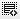1-844-696-6465 (US)        +91 77600 44484        help@dezyre.com

## make_blob() function for clustering0

Hi, I am trying to follow class examples to generate plot for clustering by making random data using make_blob() function.

``````clustering_data_X, clustering_data_y = make_blobs(n_samples=500,
n_features=5, centers=4)``````

Then to plot it i used following seaborn's function:

``````sns.regplot(x = clustering_data_X, y = clustering_data_y,
fit_reg=False)``````

and i get following error:

`ValueError: x and y must be the same size`

I understand that dimension of X (5x500)and Y (1x500) is different. I am provinding X and y as required by regplot function, then why I am getting this error?

Best, KB

EDIT:

I tried it with reg_data and ran into same issue. How do we choose which feature to take on x axis among 'n' features?0

The trouble as I understand is with the way you are trying to plot. Here is a code snippet that might help you. Go throgh it and if you need any clarity, then I can explain it.

``````from sklearn.datasets import make_blobs
import seaborn as sns
import pandas as pd
from itertools import combinations
import matplotlib.pyplot as plt
%matplotlib inline

n_features = 5
n_samples = 500
n_centers = 4
X, y = make_blobs(n_samples, n_features, n_centers)
data = pd.DataFrame(X, columns=['Feature {:2d}'.format(i)
for i in range(n_features)])
data['cluster'] = y
for i, j in combinations(range(n_features), 2):
print('Plotting Feature {:2d} vs Feature {:2d}'.format(i, j))
sns.lmplot(x='Feature {:2d}'.format(i),
y='Feature {:2d}'.format(j),
hue='cluster',
data=data,
fit_reg=False
)
plt.show()``````0

Thanks GPS. I understand it. In above example we are plotting each feature against other feature and using y-value as different color.

I was trying to replicate our class example where we did below plotting x against y :

``````import seaborn as sns
sns.regplot(clustering_data[:,0], clustering_data[:,1], fit_reg=False)``````

But i guess that worked because we only had two features. but in my example i have 5 features.

So it is correct to asume that :

• if we have two variables one x and other y ; then we plot them against each other (as we did in class)
• if we have multiple features and one target variable, then we plot features against ecah other and use y as color

What I don't understand is how do we decide which plottng method to use : regplot or lmplot ?

Best,

KB0

Plots are 2 dimensional. So only 2 features can be used at a time. So we use multiple You can use color and size of bullet to add some more values but they can become difficult to understand. This is why we use scatter matrix to understand relationship between all the features and target.

regplot vs lmplot: These perform very similar purpose and its ok to use any one of them. lmplot has the advantage that it lets you use the hue (or color) aspect for distinguishing between classes.0

Okay. This is helpful. Thanks GPS!

Click on thisicon to add code snippet.